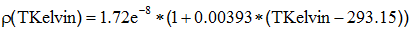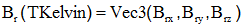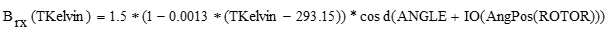# Steps of magnetic project description in Flux 2D/3D

## Presentation

The steps in the preparation of the electromagnetic Flux 2D/3D project, for a co-simulation with thermal software, are presented in this section.

## Step 0: project preparation

Project preparation (geometry, mesh, physics).

Attention: in the definition of physics, non-meshed coils in 3D and stranded coil conductors coils are not compatible with the cosimulation method. Solid conductors coils must be created .

In fact, the cosimulation exchanges only local quantities (losses densities for the magnetic project). If coil conductors or non-meshed coils are used, user has to convert the global quantities manually by dividing by the region volume.

## Step 1: Create TKelvin

The first step is to create the predefined spatial quantity « TKelvin » that will store the values of the temperature, coming from the thermal project, in each node of the electromagnetic mesh. For that, go on the menu Parameter/Quantity > Spatial quantity > New spatial quantity Tkelvin for temperature

## Step 2: Create materials with properties dependent on TKelvin

Create the materials with a predefined temperature dependent model or a spatial model whose formula depends on the TKelvin temperature and associate them to the corresponding regions.

• Example 1 : Resistivity of the winding in Copper
• Model J(E) : "Spatial isotropic resistivity"
• Formula :• Example 2 : Residual magnetic flux density of a magne
• Model B(H) : "Spatial linear magnet"
• In this case, the definition is done by a 3D vector defined by its three components in the global coordinate system XYZ1* :• With, for example :ANGLE is the angle to define Brx in the global coordinate system

IO(AngPos(ROTOR)) is the mechanical set rotation angle (this operation is a temporary workaround to take into account the movement)

*it is a current limitation not to have the choice of the coordinate system for vector definition. The passage to the coordinate system XYZ1 may require a trigonometric calculus beforehand.

Another limitation : given the limitation in number of characters, it is advisable to create the three spatial quantities Brx, Bry, Brz.

## Step 3: Open the multiphysics context

Once the project definition is over, one must create a solving scenario that will be used for the magneto-thermal cosimulation. The multiphysics context can then be opened by using this scenario. For that, go on the menu Solving > Multiphysic solving session

Remark : To open the multiphysics context in a steady state AC magnetic, one must create a void scenario (equivalent to a solving scenario with the reference values)

## Step 4: Exporting the electromagnetic nodes

Starting from the electromagnetic project, the coordinates of the electromagnetic mesh are exported to:

• DEX file for Flux-Flux cosimulation
• VTK file for Flux-STAR-CCM+ cosimulation

These files are then imported into the thermal project in order that the calculus and the temperature export could be done onto the electromagnetic mesh.

From this step, the magnetic and thermal projects have to be completed in parallel. User has to do the step 4 in thermal project in parallel to this step, and so on for the other steps.

Remark: As to the choice of position of the mechanical set, it is advisable to choose the «position of reference». This permits the software to take the nodes of the first step and to avoid problems in case of non identical motion between the electromagnetic and thermal projects.

The command is available through the menu: Parameter/Quantity > Export nodes of regions

## Step 5: Create multipoints support

In the electromagnetic project, multipoint supports are created with:

• The thermal mesh nodes for a Flux-Flux cosimulation. The losses computation and export will be done on thermal nodes. (For that, first, thermal nodes have to be exported in files (see step 4)).
• The magnetic mesh nodes for the Flux-STAR-CCM+ cosimulation

The command is available through: Coupling tools > Multi point support > New

## Step 6: Create multiphysics formula

L'entité « formule multiphysique » correspond à la grandeur ou la formule qui sera calculée et exportée. Il existe :

• Predefined multiphysics formulas:
• Joule losses : corresponding to the formula "dLossV"
• Iron Bertotti losses (with the associated information to fill)
• Iron LS losses (with the associated information to fill)
• Temperature : To be used in the thermal project
• One multiphysics formula «spatial formula» permits user to select a formula,

Remark 1: Upon creating a multiphysics formula, a spatial quantity having the same name is automatically created. This permits the storage of calculated values in order to export them into thermal.

Remark 2: A multiphysics formula equal to the sum of several multiphysics formulas will not work.

To create a multiphysics formula go on the menu Coupling tools > Multiphysics formula > New

## Step 7: Create data to export

An entity named «Data to export» permits the definition of information concerning what is being exported:

• Multipoint support where the multiphysics formula will be calculated, with the possibility of verifying if a support belongs to a region (it is advised to make this check to have the guarantee that the computation is done on the chosen region, especially on the regions border)
• The unit of length, the coordinate system, the position of the mechanical set (see step 4 above)
• The associated multiphysics formula

Each data to export corresponds to an exported file.*

*NB: the name of the data to export must be exactly the same as the name of the data to import in the thermal project.

To create data to export, go to the menu Coupling tools > Exported data > New

## Step 8: Create data to import

An entity named «Data to import» permits to define the information concerning what is being imported:

• Spatial quantity which will store the value to be imported : choose TKelvin
• Region on which the spatial quantity is imported
• The unit of length, the coordinate system, the position of the mechanical set (see step 4 above)
• The importing method: «node to node» or «by proximity». The former is chosen in the case when the thermal data is calculated on the coordinates of the electromagnetic mesh*. The latter is chosen if the thermal data is calculated on the thermal mesh**.

As explained in the previous section, the name of a data to import must be exactly the same as the name of the equivalent data to export defined in the thermal project.

*The «node to node» method may not function, if the thermal and magnetic geometric accuracies are very different.

** The «by proximity» method can be chosen in the electromagnetic project, as the thermal mesh is denser than the electromagnetic mesh. Nevertheless, it cannot be chosen in the thermal project. It is then necessary to enter a distance (in the measure unit mentioned above); this distance defines the maximum radius where the closest node can be chosen.

To create data to import, go on the menu Coupling tools > Imported data > New

## Step 9: Create cosimulation

An entity named «Cosimulation» permits the definition of information concerning the cosimulation :

• The type of cosimulation : defines the software with which Flux is coupled (it can be Flux, STAR-CCM+, Fluent or other (generic cosimulation)
• In the case of a transient magnetic, choose the type of application:
• Steady state: in this case, the time interval equivalent to an electric period is used. The averaging of the losses will be done on this interval.
• Transient: in this case, the number «n» of steps between each exchange of data is asked in order to synchronize the exchange with the thermal transient project (the export will be done at every n steps)
• The folder of exchange must be the place where both the communication files and the exported results files are located (for a cosimulation with STAR-CCM+, it corresponds to the directory containing the STAR-CCM+ simulation file)
• The relative accuracy of the convergence can be entered in the magnetic project or the thermal project, but only in one of the two. (acc. to remark in paragraph Steady state magnetic with steady state/transient thermal cosimulation). In general, it is defined in the electromagnetic project in order to verify the imported temperature convergence. The relative accuracy value can be kept by default (1%).

In the case of a cosimulation with STAR-CCM+, the convergency criteria can be defined in Flux or the user can stop manually the cosimulation in STAR-CCM+ after verifying the stabilization of the studied thermal quantity curve

• The list of data to export
• The list of data to import

## Step 10: Solve

Once all the entities are created, it remains only to launch the solving process of the cosimulation in each of the two pieces of software. For that, go on the menu: Multiphysics solving > Solving the cosimulation > New